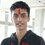# A series that came up while solving an integral

While solving problem 9 of Brilliant Integration Contest Season 1, I did the following -

\begin{align}I= \int_{0}^{1} \log \left(\dfrac{1+x}{1-x}\right) \dfrac{1}{x\sqrt{1-x^2}} \ \mathrm{d}x &= 2 \int_{0}^{1}\sum_{n=0}^{\infty} \dfrac{x^{2n+1}}{2n+1} \dfrac{1}{x\sqrt{1-x^2}} \ \mathrm{d}x\\ &=2\sum_{n=0}^{\infty} \int_{0}^{1} \dfrac{x^{2n}}{(2n+1)\sqrt{1-x^2}} \ \mathrm{d}x \end{align} Then I used the substitution $$x \mapsto \sin \theta$$.

\begin{aligned} \therefore I &=2\sum_{n=0}^{\infty} \int_{0}^{\pi/2} \dfrac{\sin^{2n} {\theta}}{2n+1} \ \mathrm{d}\theta\\ &=\pi \sum_{n=0}^{\infty} \dfrac{(2n)!}{2^{2n}(n!)^2(2n+1)} \end{aligned} The last step is due to Wallis' formula.

Now, my question is that how do we evaluate this last series?Note by Pratik Shastri
6 years, 8 months ago

This discussion board is a place to discuss our Daily Challenges and the math and science related to those challenges. Explanations are more than just a solution — they should explain the steps and thinking strategies that you used to obtain the solution. Comments should further the discussion of math and science.

When posting on Brilliant:

• Use the emojis to react to an explanation, whether you're congratulating a job well done , or just really confused .
• Ask specific questions about the challenge or the steps in somebody's explanation. Well-posed questions can add a lot to the discussion, but posting "I don't understand!" doesn't help anyone.
• Try to contribute something new to the discussion, whether it is an extension, generalization or other idea related to the challenge.

MarkdownAppears as
*italics* or _italics_ italics
**bold** or __bold__ bold
- bulleted- list
• bulleted
• list
1. numbered2. list
1. numbered
2. list
Note: you must add a full line of space before and after lists for them to show up correctly
paragraph 1paragraph 2

paragraph 1

paragraph 2

[example link](https://brilliant.org)example link
> This is a quote
This is a quote
    # I indented these lines
# 4 spaces, and now they show
# up as a code block.

print "hello world"
# I indented these lines
# 4 spaces, and now they show
# up as a code block.

print "hello world"
MathAppears as
Remember to wrap math in $$ ... $$ or $ ... $ to ensure proper formatting.
2 \times 3 $2 \times 3$
2^{34} $2^{34}$
a_{i-1} $a_{i-1}$
\frac{2}{3} $\frac{2}{3}$
\sqrt{2} $\sqrt{2}$
\sum_{i=1}^3 $\sum_{i=1}^3$
\sin \theta $\sin \theta$
\boxed{123} $\boxed{123}$

Sort by:

Have to changed the limits to obtain the consequences in using wallis formula.

- 6 years, 8 months ago

I didn't get what you are saying.. could you explain?

- 6 years, 8 months ago

You are in class 12 and you know such a high level calculus , can you give any suggestions or a recommened a book for increasing my ability in calculas@Pratik Shastri

- 6 years, 8 months ago

Well, I generally just browse the internet for interesting topics, and Wikipedia pages certainly help.

- 6 years, 8 months ago

Well, @Krishna Sharma just posted a similar question as a problem. here. It is not exactly the same but quite a bit yeah. And it has a solution too.

- 6 years, 4 months ago

I posted this long ago :P I now know how to get the result.

- 6 years, 4 months ago

Can you post the result here ?

- 6 years, 4 months ago

Well, you can use Beta function!

- 6 years, 4 months ago